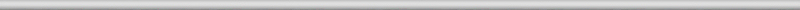## November 30, 2007

### Connectivity (or Lengths of Digital Curves, part 6)

Yes, part 6! I thought I was done with the topic (Part 1, Part 2, Part 3,…), but a couple of days ago I ran into this blog post: General connectivity (MATLAB Central) . The issue is connectivity in digital images: 4-connectivity, 8-connectivity, and other “connectivities”. The issue (adjacency of pixels) is discussed in the wiki. When I wrote this article though I did not realize that the topic is related to measuring lengths of curves. Indeed, the 8-connectivity produces curves that go only horizontally or vertically while the 4-connectivity allows diagonal edges as well. In the post the curves appear as “perimeters” of objects. More accurately, they should be called contours or boundaries of objects as the perimeter is mathematically the length of the boundary (that’s where “meter” comes from). But bwperim is the name of the standard MATLAB command for finding the boundary and we will have to live with that…

My problem is with this idea: if you are a coder it is important to make the right choice of connectivity. Let’s break this into in two statements:

1. The choice of connectivity is important.
2. The choice of connectivity is up to the coder.

The second statement reflects a general attitude that is very common - be it MATLAB or OpenCV. Important decisions are left to the coder and are hidden from the user. If something is important, I as a user would want software that produces output independent of a particular implementation. The two statements simply contradict each other.

Now, is the choice of connectivity really important?

My answer is NO.

The change of connectivity changes the boundary of the object and, therefore, its perimeter. This seems important. But we are interested of the perimeter of a “real” object, which should be independent (as much as possible) from the digital representation. This perimeter is the length of a “real” curve – the boundary of the object. We showed (in Part 3) that the relative error of the computation of length does not diminish with the growth of the image resolution. The accuracy is improved only by choosing more and more complex ways to compute the length (roughly, increasing the degree of the approximation of the curve). The choice of connectivity is determined by a 3×3 “matrix” (it’s not a matrix, it’s just a table! – another annoying thing about MATLAB). With finitely many choices the error can’t be reduced to arbitrary low. You may conceivably improve the accuracy if you can choose larger and larger “matrix” (table!), but that seems pointless…

There is another reason to think that this choice isn’t important. About that in the next post.

P.S. To clarify, every matrix is a table but not every table is a matrix (even if it contains only numbers). It is my view that tables should be called matrices only in the context of matrix operations especially multiplication. In particular, a digital image is table not a matrix.

### 2 Responses to “Connectivity (or Lengths of Digital Curves, part 6)”

1. Steve Eddins Says:

Hi,

MATLAB is widely used for algorithm development and prototyping in the area of image processing. Algorithm developers need to be aware of varying definitions of connectivity, and their tools need to provide appropriate choices. Defining neighbor adjacencies applies to many different image processing algorithms; bwperim was merely the example I chose to illustrate.

It’s true that bwperim does not compute the perimeter, and I understand why you might not like the name. Rather, it computes the set of digital image pixels that lie along the boundary, or perimeter, of a connected set of foreground pixels. Connectivity comes into play in deciding which pixels are on the boundary. Pixels are judged to be on the boundary if they are adjacent to a background pixel. Adjacency depends on the connectivity definition in use. I agree with you that this is not especially relevant to estimating the perimeter of the “real” object, but that is not bwperim’s purpose.

Finally, I think your definition of matrix is unnecessarily restrictive. American Heritage, for example, gives the mathematics definition of matrix as “A rectangular array of numeric or algebraic quantities subject to mathematical operations.” A table is “An orderly arrangement of data, especially one in which the data are arranged in columns and rows in an essentially rectangular form.” Either term seems to apply. Given my own background in discrete systems and signals, I’d really prefer something like “two-dimensional sequence,” but since I work in MATLAB, “matrix” is very convenient to use and I see no definition-based reason not to use it.

Best regards,

Steve